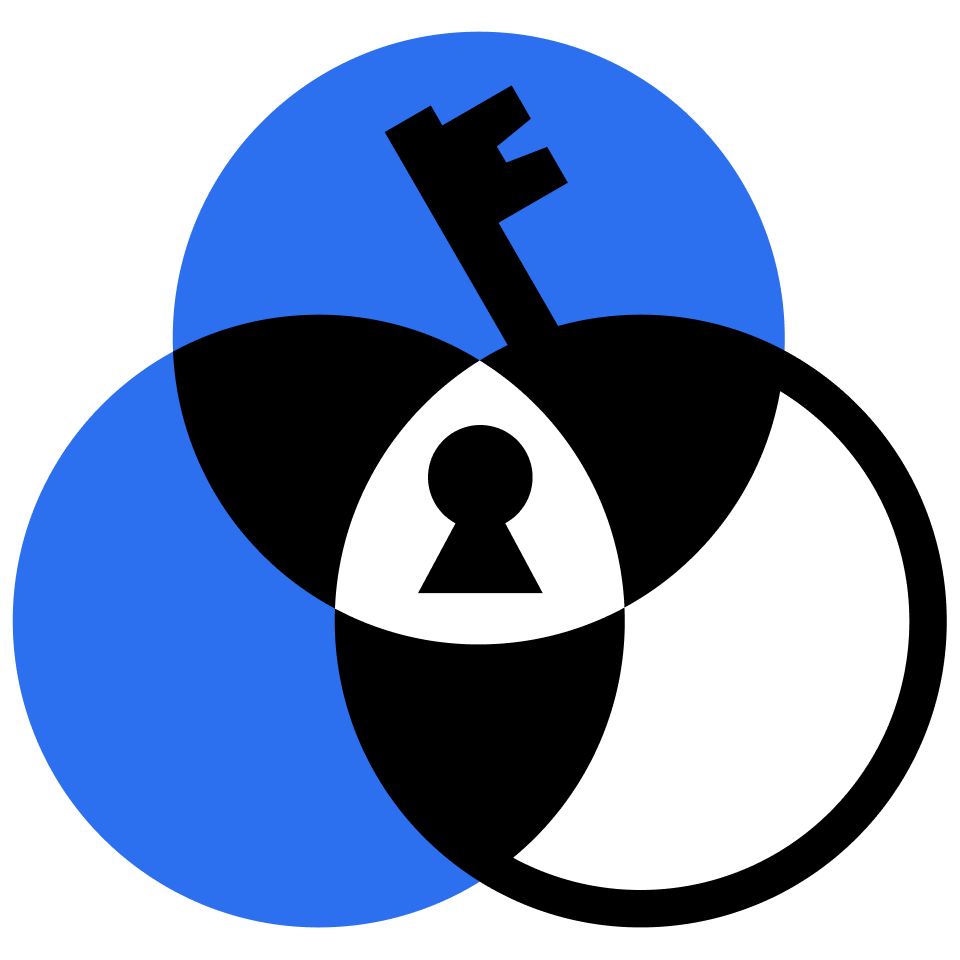## Logic II

Exercise your rationality and learn the mathematical dialects of logic.

Fallacies

Knights, Knaves, and Logic

Knights and Formal Logic

Euler and Venn Diagram Basics

All, Some, and None

Spot the Fallacies!

The Square of Opposition

And, Or, and Not

De Morgan's Laws

Truth Tables

Basic Logic Gates

Combinations

Fredkin Gates

Binary Refresher

Creating a Binary Comparator

Subtraction

Multiplication

Division

Introduction to Formal Logic

Formal De Morgan

Using Implication

Rules of Substitution

Knights and Knaves Redux

For All and There Exists

Formal Symbolization

Multiple Generality

Duals and Prenex

Quantifiers and Proof (I)

Quantifiers and Proof (II)

Functions and Identity

### Course description

Continue your adventures in logic with puzzles involving Venn diagrams and syllogisms, propositional logic, and first-order logic. By the end of this course you'll have explored the deep foundations of truth as well as applied logic to AI expert systems and linguistic analysis.

### Topics covered

• Euler Diagrams
• Venn Diagrams
• Syllogism Fallacies
• The Square of Opposition
• Binary Arithmetic
• Comparators
• Subtractors
• Multipliers
• Dividers
• Logic Symbolism
• Modus Ponens
• Modus Tollens
• Rules of Substitution
• Rules of Inference
• Proof by Cases
• Implication

### Prerequisites and next steps

You should have some experience with the logical connectives AND, OR, and NOT.

• Logic